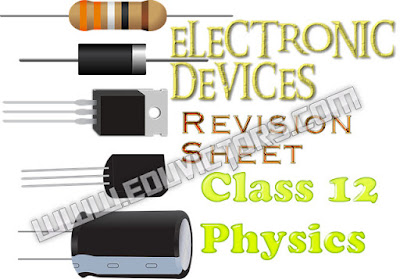## CHAPTER 9 - ELECTRONIC DEVICES - REVISION ASSIGNMENT

1 MARK QUESTIONS

1.  In a semiconductor which of the following carries can contribute to the current?
(a) Electrons
(b) Holes
(c) Both
(d) None

2. A semiconductor diode has ______
(a) One PN junction
(b) Two PN junctions
(c) Three PN junctions
(d) Four PN junctions

3. If the arrow of diode symbol is positive with respect to bar, then the diode is ______ biased

4.  The DC resistance of a diode is _______ its AC resistance.

5. If the temperature of the diode increases,then leakage current ________.

1. (c) Both
2. (a) One PN junction
3. forward
4. less than
5. increases

2 MARKS QUESTIONS

6. How is a p-type semiconductor formed? Name the majority carriers in it. Draw the energy band diagram of a p-type semiconductor.

7. How is an n-type semiconductor formed? Name the majority carriers in it. Draw the energy band diagram of a n-type semiconductor.

8. With the help of a diagram, show the biasing of a light emitting diode (LED). Give its two advantages over conventional incandescent lamps.

9. Draw a circuit diagram to show how a photo-diode is biased. Draw its characteristic curves for two different illumination intensities.

10. Give the logic symbol for an AND gate. Draw the output wave form for input wave forms for this gate.

3 MARKS QUESTIONS

11. What is rectification? How can a diode valve be used as half wave rectifier and full wave rectifier?

12. Explain how the depletion layer and the barrier potential are formed in a p-n junction diode.

13. Draw a circuit diagram for use of NPN transistor as an amplifier in common emitter configuration. The input resistance of a transistor is On changing its base current by, the collector current increases by 2 mA.
If a load resistance of is used in the circuit, calculate
(i) the current gain &
(ii) voltage gain of the amplifier

14. The output of an AND gate is connected to both the inputs of a NAND gate. Draw the logic circuit of this combination of gates and write its truth table.

15. What is a Zener diode? How it is symbolically represented? With the help of a circuit diagram, explain the use of Zener diode as a voltage stabilizer.

16. With the help of a suitable diagram, explain the formation of depletion region in a p-n junction. How does its width change when the junction is:
(i) forward biased?
(ii) reverse biased?

5 MARKS QUESTIONS

17. Explain briefly with the help of a circuit diagram how V-I characteristics of a p-n junction diode are obtained in:

(i) forward bias
(ii) reverse bias.

18. Explain the function of base region of a transistor. Why this region is made thin and lightly doped? Draw a circuit diagram to study the input and the output characteristics of n-p-n transistor in a common emitter (CE) configuration. Show these characteristics graphically. Explain how current amplification factor of the transistor is calculated using
output characteristics.

19. Draw the energy bands of p-type and n-type semiconductors. Explain with a circuit diagram the working of a full wave rectifier.

20. Explain with the help of a circuit diagram the use of an n-p-n transistor as an amplifier in common emitter configuration. Draw the input and output wave forms of the signal. Write the expression for its voltage gain.

21. What is an n-p-n transistor? How does it differ from p-n-p transistor? Give their symbols. Explain transistor action.